# Top logical reasoning questions and answers

By:

Are you seeking some quick assistance with your preparation for placement interviews? You're covered by us! Start your preparation by addressing these carefully chosen logical reasoning problems and solutions to ace the written portion of the job interview. In this article, we covered a sample of logical reasoning questions and answers so as to help you master the concepts.

## What is Logical Reasoning?

The subject of logical aptitude tests is always changing. In the study of logical thinking, numerous theories and notions with logical problems are discussed, and their justifications are derived. It borrows concepts from numerous fields and evaluates how well the person applies the logic of those fields to the provided approach.

In other words, logical reasoning will evaluate a person's understanding of each subject individually. There is only one method for learning this topic. To practise, that is. As you practise, you will gain a deeper understanding of the subject's fundamentals and develop logical reasoning skills that will serve you well throughout your life. Logical reasoning is divided into two parts. They are:

• Verbal logical reasoning
• Non-verbal logical reasoning

### Coding and Decoding Questions

A technique called coding is used to encrypt text or integers using precise patterns and set rules. It's also known as encryption in a more technical sense. Additionally, it is frequently used on all messaging systems to safeguard the context of user communications..

Take a look at the coding problem we have here.

Letters A to Z are coded using the cells in diagram I and sectors in diagram II. Now, the first letter in each cell is coded by its shape; however, the second letter includes a dot in it. Using this instruction, answer the question given below.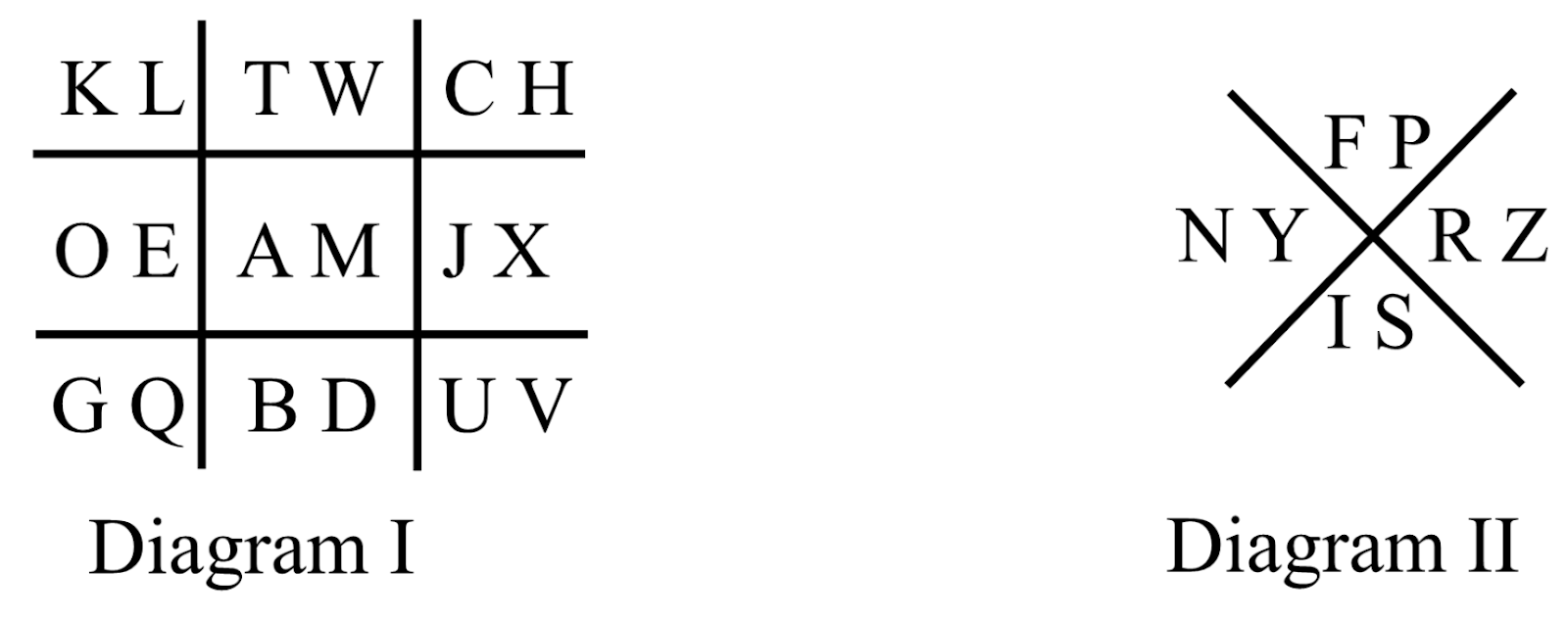How will STEAM be coded?

Solution: The diagram is given as below,Now, STEAM can be coded as,

As S, is the second alphabet in the figure, so it will be represented as,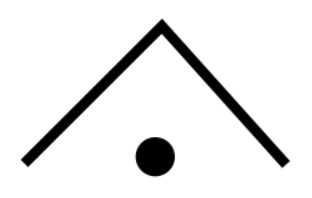T is the first letter, so it will be represented as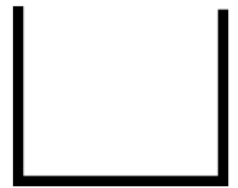E is the second letter, so it will be represented by a dot,A is the first alphabet, so it will be represented as,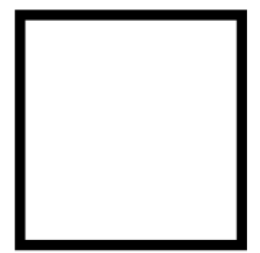M is the second alphabet, so it will be represented as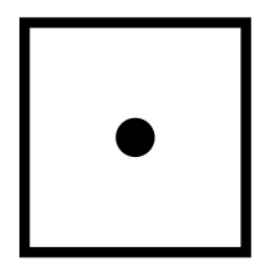Hence, STEAM can be coded as### Series Completion Questions

The candidate must identify a pattern in a given set of numbers or letters in this part. The next step is for them to either select a solution that applies the same logic in a series or identify the term that violates the logic of sequence. Let's take a look at a series questions.

Understand the pattern and complete the series.

5, 16, 49, 104, ?

Solution: The given series is,

5, 16, 49, 104, ?

Now, if we observe the series, we can see the pattern followed by the numbers is,

5+(11×1)=16

16+(11×3)=49

49+(11×5)=104

104+(11×7)=181

Hence, the required answer is 181.

### Direction Sense Questions

Questions about direction sense frequently involve a subject going in a series of directions that involve numerous turns. These logical reasoning tests are designed to evaluate a candidate's pen-and-paper direction perception skills.

If ‘South-east’ is called ‘East', ‘North-west' is called ‘West’, 'South-west' is called ‘South’ and so on. What will ‘North’ be called?

Solution: After carefully observing the given question, it can be concluded that the South-East in the question is called East by rotating 45 degrees anti-clockwise, similarly the North-West is called South by rotating 45 degree anti-clockwise and the sequence continues further. Based on the information in the question, it is shown by a different diagram below.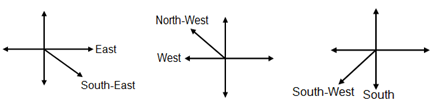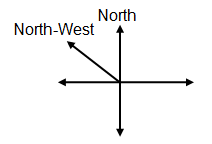Hence it is clear from the diagram that North is called North-West by rotating 45 degrees  anti-clockwise.

### Seating Arrangement Questions

These logical thinking problems need you to arrange the people or things in accordance with the requirements stated in the question. You must be aware of the seating arrangement, the person's direction—for example, whether they are facing inside or outward—in order to respond. Look at the following seating arrangement problem now.

Five persons A, B, C, D and E are sitting in a row facing you such that D is on the left of C and B is on the right of E. A is on the right of C and B is on the left of D. If E occupies a corner position, then who is sitting in the center?

Solution: According to the information given in the question, we get that

Five persons A, B, C, D and E are sitting in a row facing me. It implies that if I am at the origin (0, 0) of XY plane, then the five persons will be facing towards the south. Now, analyze the given statement.

E occupies a corner position. As B is on the right of E, so E must be on the right side as shown below.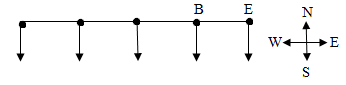Now, B is on the left of D, which means D is on the right of B. As D is on the left of C, it implies that C is on the right of D. A is on the right of C. Now, draw the diagram accordingly.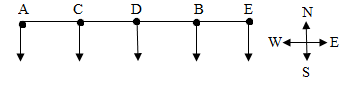From the above diagram, it is clear that if E occupies a corner position, then D is sitting in the center.

### Blood Relationship Questions

The questions asked in these logical reasoning tests used to be simpler and more statement- or dialogue-based, but as the competition has grown, so has the variety of problems. Questions about blood relationships can now be found in a variety of ways, including jumbled descriptions, relational puzzles, and coded relations. Consider the following logical reasoning tests based on blood relationships.

Danish is the brother of Ravish. Rekha is the sister of Antarish. Ravish is son of Rekha. How is Danish related to Rekha?

Solution: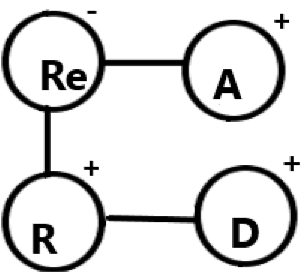In the given figure, D represents Danish, R is Ravish, Re is Rekha, and A is Antarish.

According to the question, D is the brother of R. Re is the sister of A. R is the son of Re, so his brother D will also be her son. A will be the maternal-uncle of R and D. Hence, Danish is son of Rekha